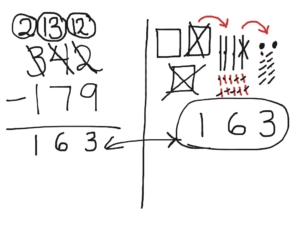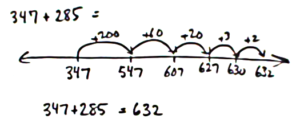# Top 5 Things to consider when teaching 3rd grade students about adding or subtracting with place value understanding!!

Here are the Top 5 things to consider when teaching 3rd grade students about adding and subtracting multi-digit numbers with place value understanding:

1. Student’s prior knowledge from 2nd grade and how it relates to 3rd grade standards

Students in 2nd grade were expected to…
• Fluently add and subtraction within 100 using strategies based on place value
• Add up to four two-digit numbers using strategies based on place value
• Mentally add/subtract 10 or 100 to a given number between 100-900
• Explain why addition and subtraction works

Students in 3rd grade are expected to fluently add and subtraction within 1,000 using strategies and algorithms based on place value.

2. Use of models to build conceptual understanding

Though students have learned addition and subtraction with a variety of strategies and models before they get to you in 3rd grade, it’s important to still encourage the use of models to deepen the conceptual understanding of what addition and subtraction is with larger numbers. This will encourage the use of place value language, which is necessary in understanding the process of addition and subtraction of multi-digit numbers within 1,000. Models may include use of base ten blocks, quick pictures or number lines. As you connect and compare these different strategies by posing questions to students, the understanding of addition or subtraction will deepen.

Base ten blocks model example:Base ten Quick Picture Example:Adding with a Number Line Example:3. Importance of explaining the addition or subtraction process with place value language

As students create models, solve with algorithms or invented strategies, it’s important to encourage the use of place value vocabulary or language to describe the steps taken to solve. This ensures that they will have a deep conceptual understanding of addition or subtraction and not just the procedure of solving a problem.

For example a student may solve the problem 216 + 372 by describing, “I added the six ones and 2 ones, so now I have 8 ones. Then I added the 7 tens and 1 ten to get a total of 8 tens. Last I added the 2 hundreds and 3 hundreds to get a total of 5 hundreds. With the 5 hundreds, 8 tens and 8 ones I have a total of 588.” This is much different than having a students say, “First I added the 2 and 6 to get 8. Then I added the 7 and 1 to get 8. Then I added the 3 and 2 to get 5.” The second explanation may create some common misconceptions about what each digit really represents and how it creates the value of the solution.

4. Presenting problems in context with story problems to encourage students to “choose an operation”

Understanding the structure of addition and subtraction is one piece to the puzzle. The other big piece is to have students determine whether they are adding or subtracting with a given story problem or scenario. In order for this to happen you pose problems, explore and share thinking about what actions in the story problem told you to add or subtract. (AVOID KEY WORDS AND FOCUS ON KEY ACTIONS). This can also be done by having students complete a problem sort by:
• Give multiple story problems on index cards/slips of paper/etc.
• Have students justify whether they would use addition or subtraction to solve and why (may include directly modeling the problem to prove their thinking)
• Place each problem into the addition or subtraction pile to show their choice

There are even some problems where they could use either addition or subtraction, depending on how you look at the problem. The most important thing is that students explain why they chose the operation they did and how they will use that operation to solve the problem correctly. For example: Tony had 715 apples total. He started with 342. Then he and his friends picked some more together. How many apples did Tony and his friends pick together?” It’s important to have a balance of the understanding of how addition/subtraction works and how to use the operations in real-world problem solving scenarios.

Students also need to break down the problem to make sense of it with numbers. They also need to determine how the numbers they are using to solve the problem relate back to the original story problem. This can be encouraged by posing questions like, “How does this number relate to the problem? How does your expression match the actions in the problem?” (This is also known as contextualizing and decontextualizing).

5. Expose 3rd grade students to a variety of problem types

It’s important that students see various problem types to prepare them for the real world. Not all problems will be presented the same way and they need to be prepared to apply the problem solving strategies they learned with any problem structure they encounter.

Problem types are listed in this Common Core Chart below:To reinforce addition of numbers to 1,000 visit https://goo.gl/Uanlr2. To reinforce subtraction of numbers to 1,000 visit https://goo.gl/fUwWhg.

Another great website to encourage the use of base ten models with the “base blocks” virtual manipulatives listed to solve addition and subtraction problems is: http://nlvm.usu.edu/en/nav/grade_g_2.html.